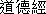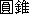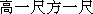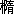Previous page || Next page || First page || References || Chinese text

Click on any figure to see it enlarged in a separate window.

## 6. Translation of the derivation

(Translation of Jiuzhang suanshu [1963, 167-168]. The Chinese text is reproduced in Section 8.)

### [6.1. The etymology of the term yangma]

The shape [called] yangma is one corner of a fangzhui. [See Section 3 above.] A corner of a hip-gabled roof [sizhu wu] is called a yangma.

Not much is known of architectural terminology in Liu Hui's time, but in the Song the term yangma meant a hip-rafter in a hip-gabled roof.

### [6.2. The case of equal dimensions]

In the following see Figure 6. Certain phrases here are referred to later in the text. These I have marked (1), (2), etc.Suppose the breadth, length, and height are each 1 chi. Multiplying these together gives the volume of a cube [with the same dimensions], 1 [cubic] chi. (1) Dividing the cube [ABCDEFGH] slantwise [along the plane of BCEH] gives two qiandu [BCDAHE and BCFGHE]; (2) dividing [one of the] qiandu [e.g., BCDAHE] slantwise [along the plane of ACFH] gives one yangma [CADEH] and one bienao [CABH]. The yangma occupies 2 and the bienao occupies 1: this is an unchanging proportion. Fitting together two bienao makes one yangma, and fitting together three yangma makes one cube. Hence the division by 3. If this is verified using blocks, the situation is clear. Cutting all of the yangma gives a total of six bienao. Looking at the pieces, it is easy to understand that the shapes correspond.

It is not completely trivial to "understand that the shapes correspond, for three of the bienao are mirror-images of the other three.

### [6.3. The general case]

(3) If the block is long or short, or broad or narrow, so that [the sides of] the cube are not equal, it can still be cut into six bienao. Their shapes are not the same, but the number [i.e., six] which appears is the same, and their volumes are in fact equal.

See Figure 7a. When the box ABCDEFGH, with dimensions a, b, c, is cut into six bienao as described above, the result is the three non-congruent bienao FADC, FEDC, and FABC (Figures 7b, 7c, and 7d) and their respective mirror images CHGF, CBGF, and CHEF. Each of the six has the same dimensions, a, b, and c, and the volume of each is 1/6 abc. But Liu Hui has not yet proved this fact.
(4) When the bienao have different shapes, then so do the yangma.
See Figure 7a. The six bienao can be put together to form three yangma as follows: FADC with FABC to form FABCD (Figure 7e),
FEDC with CHEF to form FEDCH (Figure 7f),
CHGF with CBGF to form FGBCH (Figure 7g). These yangma have the same dimensions, but they are not congruent. There is one other way of fitting the bienao together to form yangma (FADC with FEDC, FABC with CBGF, and CHEF with CHGF), and in this case again the yangma are not congruent.
When the yangma have different shapes, then they cannot be compared [chunhe]. When they cannot be compared, then it is difficult to do it [i.e., derive the formula].

### [6.4. An obscure digression]

The following passage seems to be an additional comment on the preceding argument.
Why is this? "Dividing the cube slantwise gives [two] qiandu" [abbreviated quotation of (1) above]; here the division is necessarily in halves. "Dividing [one of the] qiandu slantwise gives [one] yangma [and one bienao]" [abbreviated quotation of (2) above]; here again the division is necessarily in halves. One [division] is vertical, and one is horizontal.
A way to make sense of this passage is as follows. See Figure 7a. If the box ABCDEFGH is cut first on the plane of FDCG and then on the plane of ACHF, then each of the cuts divides the volume of the box in halves; these cuts might reasonably be described as "vertical" and "horizontal," respectively.
The result of these cuts is two yangma, FEDCH and CBAFG, and two bienao, FADC and CHGF. Note that the two yangma are congruent mirror images, and that the two bienao are congruent mirror images.
Suppose a yangma is on the inside of a division, and a bienao is on the outside. Even if "the block is long or short, or broad or narrow" [reference to (3) above], there is still this constant proportion of the divisions. It is only through this that one knows that the "different shapes'' [reference to (4) above] are also equal.
The intention of this passage might conceivably be to show that mirror image bienao or yangma are equal, since for example,
FEDCH + FADC = 1/2 ABCDEFGH,
FEDCH + CHGF = 1/2 ABCDEFGH,
but this is unlikely, since Liu Hui does not elsewhere seem concerned about the problem of mirror images.

### [6.5 division of the yangma and bienao into smaller pieces]

Here begins the derivation of the formula in the general case. The argument is expressed in terms of the case of equal dimensions, and it is necessary for the reader to extend the argument to the general case.To make a bienao with breadth [a], length [b], and height [h] each 2 chi, use two qiandu and two bienao blocks, all of them red.

Figure 4 shows how the blocks are fitted together.
To make a yangma with breadth [a], length [b], and height [h] each 2 chi, use one cubical block, two qiandu blocks, and two yangma blocks, all of them black.
Figure 5 shows how the blocks are fitted together.
Joining together the red and black blocks to make a qiandu, the breadth, length, and height are each 2 chi.
This qiandu is shown in Figure 8.The following is probably corrupt; my translation is speculative and involves some emendations to the text (see notes 10-12). Whether or not this translation is completely correct, the interpretation of the mathematical sense of the passage is fairly certain.

Then divide [xiao]  the breadth in the middle and divide [fen] the height in the middle.
The two divisions are on the planes of HJMK and KMPN.
Fit the red and the black qiandu together, in each case forming a cube with height 1 chi and sides [each] 1 chi. 
The red qiandu AGIJML and ILMJCP are fitted together, and the black qiandu FQONKL and CPORIL are fitted together. Two cubes are thus formed, one red and one black.
In the general case, these qiandu do not fit together. However it is clear that in each case the sum of the volumes of the two qiandu is equal to that of a box with dimensions 1/2 a, 1/2 b, 1/2 h.
Each division then contains one bienao and one yangma. 
The "divisions" are ABHJMK and KMPNFE in Figure 8. Since the qiandu in these divisions have been dealt with, what is left is one bienao and one yangma in each.
Each of the remaining items is composed of [blocks with the same form as] the original objects.
The two "items" are the two qiandu BGIHKL and EQOPML, each of which is composed of one red bienao and one black yangma.
From this point on there are no major difficulties with the text.
These fit together to form a cube.
In the general case these pieces fit together to form a box with dimensions 1/2 a, 1/2 b, 1/2 h.
Thus cubes [formed of blocks] which are different [from the original bienao and yangma] occupy a proportion of 3, and cubes [formed of blocks] which have the same form occupy a proportion of 1.
The situation is now as follows.
Black: 1 cubical block, and 1 cube formed of two qiandu blocks;
Red: 1 cube formed of two qiandu blocks;
Red and black: 1 cube formed of two red bienao blocks and two black yangma blocks.
In the general case,
Black: a volume of 1/4 abh;
Red: a volume of 1/8 abh;
Red and black: 2 red bienao with dimensions l/2 a, 1/2 b, 1/2 h; 2 black yangma with dimensions 1/2 a, 1/2 b, 1/2 h, ; total volume l/8 abh.
Thus the original 2 x 2 x 2 bienao and yangma have been divided in such a way that three-quarters of the total volume consists of objects whose volumes are known, and one-quarter consists of l x l x l bienao and yangma. The ratio of the volumes of the known objects is red:black = 1:2, and this ratio holds also in the case of arbitrary dimensions .
Even if the cube is elongated, and the blocks change [accordingly], there is clearly a constant situation.

### [6.6. Carrying the operation to the limit]

The commentator Li Huang(d. 1812 [Qian 1964, 296]) could make no sense of the following and considered it to be corrupt [Qian 1963, 169, n. 1]. Actually the text is fairly clear once one understands what Liu Hui is trying to do.
Of the remaining numbers [i.e., the volumes of the pieces resulting from the above manipulations], those which can be definitely determined are separated into one and two parts [one red cube and two black cubes]. Thus, it has been determined that the ratio [of the numbers which can be definitely determined] is 1 to 2. In terms of principle, how could this be arbitrary? To exhaust the calculation, halve the remaining breadth, length, and height; an additional three-quarters can thus be determined. The smaller they are halved, the finer [xi] are the remaining [dimensions]. The extreme of fineness is called "subtle" [wei]; that which is subtle is without form [xing]. When it is explained in this way, why concern oneself with the remainder?
On this passage see Section 5 above.
Exhausting the calculation is called "calculating with the essence"; one "does not use calculating-rods to calculate it". [Cf. Daode jing, Chap. 27: "The skilled calculator does not use calculating rods."]

### [6. 7. Justification for this concern with impractical matters]

The object [called] bienao has no practical use; the shape [called] yangma can be long or short, or broad or narrow. Nevertheless, without the bienao there is no way to investigate the number [i.e. volume] of a yangma; and without the yangma there is no way to know [the volumes of] such things as zhuiand ting. These are primary in practical application.

"Zhui and ting" is a condensed form referring to four geometric figures: fangzhui(see Section 3 above),
yuanzhui(cone),
fangting(see sections 3 and 4 above),
yuanting(truncated cone).

I am grateful to Else Glahn for explaining this matter to me. Documentation will be found in her forthcoming book on the Song architectural manual Yingzao fashi.

This passage gives difficulty. It would be most natural to take chunheto mean "fit together precisely," so that the translation might be, "When the yangma have different shapes, then they cannot be fitted together precisely. When they cannot be fitted together precisely, . . . " There are two reasons why this cannot be the correct interpretation: (1) the yangma can in fact be fitted together precisely to form a box; (2) this is not the problem. Liu Hui's problem is that the three yangma are not congruent, so that it is not proved that their volumes are equal. The sentence must deal with the volumes of the yang-ma, and therefore I tentatively translate chunhe with the vague word "compare."

She yangma wei fen nei, bienao wei fen wai, qi sui . . .Qian Baocong's emendation is unnecessary.

The word xiaohas a variety of meanings, including "to compare" and "to imitate." I interpret it here as "to divide," primarily because this is the only interpretation which makes sense in the context. Note that the word is used parallel with the word fen, which definitely has this meaning.

Here I emend gao er chi fang er chito gao yi chi fang yi chi, i.e. I take the dimensions of the cube to be 1 x 1 x 1 instead of 2 x 2 x 2.

Here I emend mei er fen bienao ze yi yangmato mei fen ze yi bienao yi yangma. Regardless of whether this emendation is correct, the original sentence is almost certainly corrupt. The only way to make sense of it is to take fen as a measure word, so that the sentence would be translated, "Each two bienao make one yangma." Not only is this sentence pointless here, but since there are only two bienao in the situation at hand, the word "each" is not necessary.

Accepting the variant duan instead of qi.

Reading tuofor sui. Another example of this substitution is in Jiuzhang, p. 166, line 11.

Previous page || Next page || First page || References || Chinese text